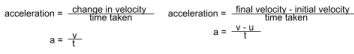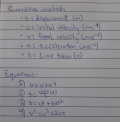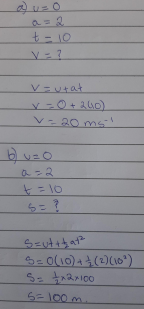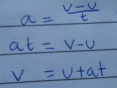# Accelerated Motion## Accelerated Motion

2.1: MEANING, CALCULATION AND UNITS OF ACCELERATION

● Acceleration: rate of change of velocity (vector quantity, since it depends on velocity, which is also a vector). Measured in ms-2 or m/s2● Since velocity is a vector quantity, if the direction of an object’s motion changes, so does its velocity. Thus, the object is accelerating

2.2: DEDUCING ACCELERATION

● A velocity-time graph can show how the velocity of an object changes over time:● The gradient of a velocity-time graph shows the acceleration of the object. The steeper the gradient, the larger the magnitude of the acceleration.

● NOTE: when given a graph, read the question or axes very carefully, so that you know if you are dealing with a displacement-time or velocity-time graph. Most times, the two may look the same, but they are telling you two very different things

2.3: DEDUCING DISPLACEMENT

● To deduce the displacement of an object from a velocity-time graph, simply calculate the area under the line. Consider the below example:

A car starts from rest and reaches a speed of 27 ms-1 after 12 s. After this, the

car travels at a constant speed of 27ms-1for another 20 s. Find:

a) The acceleration of the car in the first 12 s

b) The total displacement of the car

To solve this, it is a good first step to draw a rough sketch (if a diagram is not

already provided), and note down the values given and those to be found. This

helps you decide which equations to use (when applicable):

Notice you have the initial and final velocities of the car, as well as the time taken for the car to move from rest to the final velocity. This gives you everything you need to solve part (a):

For part (b), using the area under the line, either one of the below two methods can be used:

● For more complicated graphs (e.g. when the graph is a curve), a suitable method could also be counting the squares under the curve on the diagram. When doing this, however, it is important to check the scales on the axes to see what 1 unit in either direction represents (e.g. 1 unit = 1 s on the time axis)

2.4: MEASURING AND DETERMINING VELOCITY AND ACCELERATION

Cars have accelerometers, which are small devices that measure large accelerations and decelerations. These have two sets of interlocking metal “teeth”, which move together in the event of a crash, producing a voltage which sets off the airbag.

● Acceleration and velocity can be measured in the laboratory using experiments such as light gates, ticker-timers and motion sensors (lack precision since velocity is determined by changes in position and acceleration is therefore determined by changes in velocity)

2.5: EQUATIONS OF MOTION

● There are four equations of motion that can be used to find different quantities:● These equations can only be used when the object is moving in a straight line and/or with constant acceleration

● When solving questions requiring equations of motion, it is good practice to write down the quantities given and the quantity asked to find. Consider the following example: A car is initially stationary. It has a constant acceleration of 2.0ms−2. Calculate the following:

a) the velocity of the car after 10 s

b) the distance travelled by the car at the end of 10 sc) the time taken by the car to reach a velocity of 24ms−1

To solve these three questions, as mentioned, first write down the given

quantities and then the quantity asked for. Then choose a suitable equation from the four, and solve for the required quantity:

2.6: DERIVING THE EQUATIONS OF MOTION

● Equation 1:

Rearranging the formula for constant acceleration to make v the subject of the formula gives equation 1:● Equation 2:

The average velocity of an object can be found by halving the sum of the initial and final velocities, giving:

Rearranging the equation for velocity to make displacement the subject gives the following:

Using average velocity in the above equation gives the second equation of

motion:

● Equation 3:

Equations 1 and 2 can be used to derive equation 3:

● Equation 4:

Equations 1 and 2 can also be used to derive equation 4:

2.7: UNIFORM AND NON-UNIFORM ACCELERATION

● The equations of motions explained so far only apply to situations where the acceleration of the object is constant (uniform).

● In cases of non-uniform acceleration, the velocity-time graph has a curve (see Section 2.2). To find the acceleration at a certain point of time, the following steps must be taken: 1. Mark a point on the line corresponding to the time value.

2. Draw a tangent through that point, following the curve as closely as possible for the best accuracy

3. Calculate the gradient of the tangent. This gives the acceleration of the object at that point in time

● To find the area under a graph showing non-uniform acceleration, count the squares under the line. Keep in mind that this may not give an accurate result, and remember to check both axes to make sure what 1 unit in either direction represents!

2.8: DEFINING AND DETERMINING ACCELERATION UNDER GRAVITY ● Acceleration of free fall: acceleration of an object due to Earth’s gravitational field (= 9.81 ms-2

● g can be measured in the laboratory through various experiments such as light gates, ticker-timers and electronic timers

2.9: PROJECTILE MOTION

● When an object is travelling downward in a field of gravity, it has an acceleration equal to g. When it is travelling upward, it has an acceleration equal to -g, since it is travelling in the direction opposite to the direction which gravity acts in

● An object’s horizontal motion is not affected by gravity, so in the absence of air resistance, its horizontal velocity is constant

● Velocity has a horizontal and vertical component. Both must be considered individually, as they are independent of each other.

● For any given velocity, the vertical and horizontal components are calculated as follows:

2.10: UNDERSTANDING PROJECTILES

● As mentioned earlier, an object travelling upwards is considered to have an acceleration of -g, since it is travelling in the direction opposite to the action of gravity. This means that all objects travelling upwards are slowing down, and will eventually have a final speed of 0 ms-1

● The equations of motion can be used to solve questions relating to objects travelling upwards and downwards in a gravitational field, because they obey the conditions mentioned previously.

● Let us first look at an object travelling vertically up and down. Consider the below example:

A boy standing on the roof of a building 10 m tall throws a ball into the air. It has an initial speed of 15 ms-1. Calculate:

a) the maximum height reached by the ball.

b) the time it takes for the ball to hit the ground

To solve this, first write down all the values given in the question, and those asked for:

Then, choose the appropriate equation of motion and solve for the missing value:

However, that is not the last step! The question asks to find the maximum height reached by the ball. Since the ball starts its journey 10 m in the air, this is added onto the height found to give the maximum height:

Notice the answer has been rounded up to two significant figures. Though this may seem strange, it is good practice. A general guideline to follow is this: if the question specifies the number of significant figures to be used, follow the question. If not, round your final answer to the number of significant figures shown in the data given (in this case, two).

For part (b), an important thing to note is the question asks the time taken for the ball to hit the ground. To solve this, first understand that the displacement of the ball when it hits the ground is -10 m. This is because when the displacement is 0 m, it has arrived back to the boy’s hand. However, the boy is still 10 m above the ground, since the height of the building is 10 m. So, the total displacement is 10

m below the starting point, which is -10 m. With this information, the question is solved as such:

● In the above example, the object travelled up and down, so the horizontal distance travelled by it remained 0 m. Thus, its horizontal velocity also remained 0 ms-1. ● Now let us look at a situation where an object has both horizontal and vertical velocity, and travels distances in both the horizontal and vertical directions. Consider the below examples:

Example 1: A boy at the edge of a cliff 50 m above ground level fires a stone from a slingshot horizontally at a velocity of 10 ms-1. Calculate:

a) the time taken for the stone to reach ground level

b) the distance of the stone away from the cliff after falling to the bottom

To solve part (a), it is important to note that the velocity used is not 10 ms-1! That is the stone’s horizontal velocity. Its initial vertical velocity is 0 ms-1, since it is fired horizontally. With this in mind, we begin by noting down the known and asked quantities:

We can use the equations of motion in this situation, since the stone has a constant acceleration (g). Choosing the appropriate equation given the quantities we have, we solve it as such:

Now, since we have the time taken for the stone to fall to ground level, we can solve part (b). Before this, however, it is important to note the following: the horizontal velocity of the stone stays constant throughout its journey, since it is independent of gravity’s effects. Thus, we can use the equation for constant velocity to solve the question:

Example 2: During a football match, the goalie clears the ball from his goal post by kicking it away with a velocity of 20 ms-1 at an angle of 30° to the horizontal. a) How long will the ball take to come back down to the pitch? b) How far away must a player stand to receive the ball?

To solve this question, our first step should be to resolve the velocity of the ball into its horizontal and vertical components. This will help us use the correct values in out equations:

To solve part (a), it is important to note that we must consider the ball’s vertical displacement. In this situation, it is 0 m, because the ball travels upwards, peaks, and then travels the same vertical distance downwards to come back to the pitch. Thus, we have the following values:

Again, since the ball has a constant acceleration (-g), we can use the equations of motion. Choosing the appropriate one, it is solved as such:

Because the resulting equation is a quadratic, we get two solutions. However, the correct answer is t = 2.04 s, because t = 0 s is when the ball starts its journey!

To solve part (b), we must now consider the ball’s horizontal motion, to find the horizontal distance travelled. As before, the horizontal velocity of the ball stays constant throughout the journey, as it is unaffected by gravity. Thus, we can use the equation of constant velocity to solve for the horizontal distance travelled: